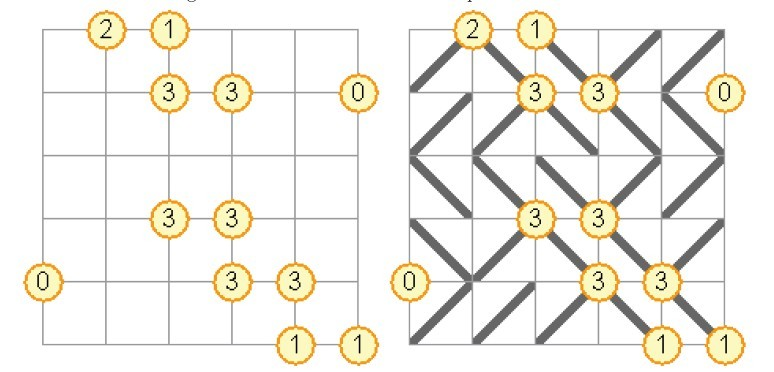# 2695. Gokigen Naname

Gokigen Naname is a Japanese puzzle game played on a square grid in which numbers in

circles appear at some of the intersections on the grid.

The objective is to draw diagonal lines in each cell of the grid, such that the number

in each circle equals the number of lines extending from that circle. Additionally, it is

forbidden for the diagonal lines to form an enclosed loop.The first figure shows the start position of a puzzle. The second figure shows the solution to the same puzzle. A Gokigen Naname puzzle always has exactly one solution.

### 输入格式

The first line of the input contains a single integer n (2 <= n <= 7), the number of cells along each of the sides in the square grid. Then follow n + 1 lines containing the contents of the intersections of the grid cells. Each such line will contain a string of n+1 characters, either a digit between 0 and 4, inclusive, or a period (‘.’) indicating that there is no number at this intersection (arbitrarily many lines may connect to it).

### 输出格式

The output should contain n lines, each line containing exactly n characters. Each

character should either be a slash or a backslash, denoting how the corresponding grid

cell is filled.

### 样例

Input
3
1.1.
...0
.3..
..2.
5
.21...
..33.0
......
..33..
0..33.
....11

Output
//
\\
/\/
/\\//
//\\\
\\//
/\\/
///\\


4 人解决，7 人已尝试。

4 份提交通过，共有 35 份提交。

8.2 EMB 奖励。